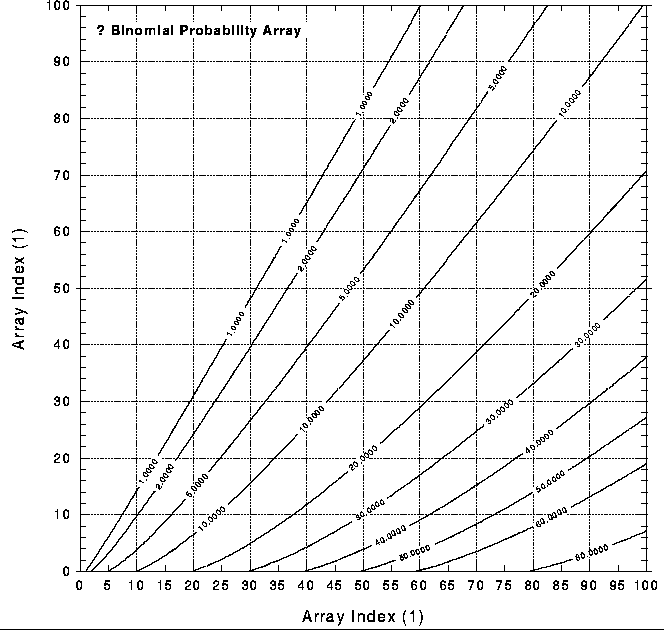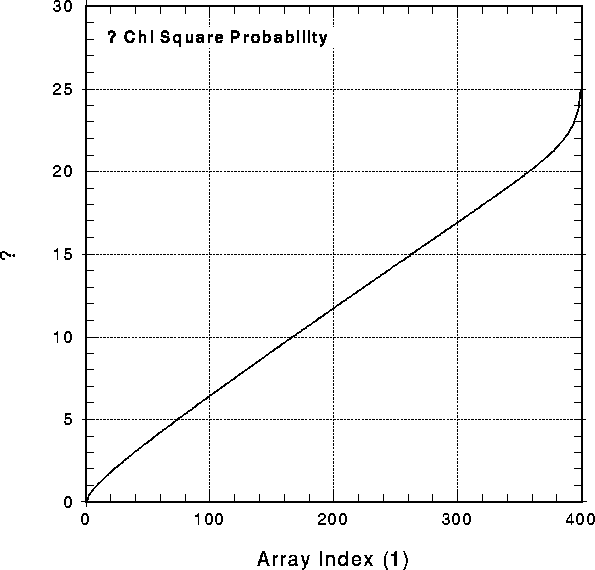Next: References Up: Input Files for Standard Previous: G.2 Files Containing Spectral

# G.3 Probability Tables

SASS uses statistical test to classify the analysis results. The binomial and chi2 distributions are stored in the files v\$manpar:pbin.bid and v\$manpar:pchsq.bid, respectively. Both distribution are plottet from the content of these files in Figure G.8 and Figure G.9.Figure G.8:   Binomial Probability. The basic information was taken from file v\$manpar:pbin.bid.Figure G.9:   Chi Square Probability. The basic information was taken from file v\$manpar:pchsq.bid.

If you have problems/suggestions please send mail to rosat_svc@mpe-garching.mpg.de